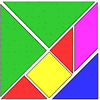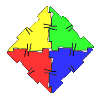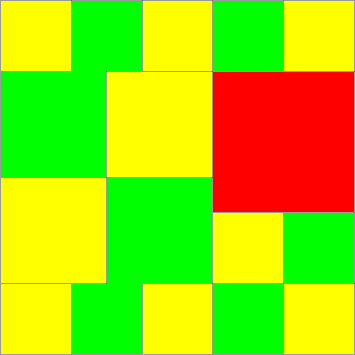#### You may also like### Tangrams

Can you make five differently sized squares from the interactive tangram pieces?### Geoboards

This practical challenge invites you to investigate the different squares you can make on a square geoboard or pegboard.### Polydron

This activity investigates how you might make squares and pentominoes from Polydron.

# Tiles on a Patio

## Tiles on a Patio

Imagine that round the back of where you live is a piece of ground that your family want to change. Instead of grass or plants growing there you want to have a patio. To prepare to change it the ground is dug up and levelled, it is then measured and found to be a square $10$ by $10$ [this could be feet, metres or yards it does not really matter for this challenge]. You then go to a large store that sells tiles to go on the ground. To your surprise you find that they sell tiles in ten different sizes; $1$ by $1$, $2$ by $2$, $3$ by $3$, etc. up to $10$ by $10$. You decide to go back home for a while and work a few ideas out.

You start by drawing a $10$ by $10$ square; and then you think about what sizes of tiles to use to cover it.

If you use $1$ by $1$ tiles then you find [or know already!] that you will need $100$ of them. So your shopping list would look like :-

$100$ tiles @ $1$ by $1$ > Total number of tiles $100$If you use $10$ by $10$ tiles then you find [or know already!] that you will need 1 of them. So your shopping list would look like :-

$1$ tiles @ $10$ by $10$ > Total number of tiles $1$

If you use $2$ by $2$ tiles then you find [or know already!] that you will need $25$ of them. So your shopping list would look like :-

$25$ tiles @ $2$ by $2$ > Total number of tiles $25$

You could of course use a mixture of different sized tiles.

If you use $12$ tiles $2$ by $2$ and $4$ tiles $3$ by $3$ and $1$ tile $4$ by $4$ your shopping list would look like :-

$12$ tiles @ $2$ by $2$
$4$ tiles @ $3$ by $3$
$1$ tile @ $4$ by $4$ > Total number of tiles $17$So you could, with these examples use $1, 17, 25$ or indeed $100$ tiles.

Your challenge starts by asking you to try to find lots of different numbers of tiles that could be used to cover this $10$ by $10$ patio.

You could think about the artistic appearance of it as well if you like. However all the patio must be covered and no cutting any of them to make different sizes or shapes ... they're actually very, very hard to cut anyway.

You could now think about these kinds of questions and perhaps you would think of other questions of your own to pursue.

1. Are there some numbers of tiles used that actually are made up of different mixtures of tiles? [For example in our one that used $17$, could you get to use just $17$ tiles but they require a different shopping list from the one above?]
2. Are there some numbers between $1$ and $100$ that cannot be used as the total number of tiles required?
3. Is there some system for getting new examples that use different numbers of tiles?

You might then think about how all this might or might not change if the patio had been $9$ by $9$ [$8$ by $8$, $7$ by $7$ etc ...]

Well good luck, and if you're artistically inclined I guess that you may have some results that would look really good displayed. Since you're sitting at a computer it may be that you could use a "draw/art" package to do all the work on the screen for you. But have a break from the screen every hour ... your body deserves it!

### Why do this problem?

Working on this investigation provides many opportunities to speak with youngsters to discover how they are going about generating new ideas. The activity encourages growth in problem solving, spatial awareness and number awareness.

### Possible approach

If you are doing this with a whole class, then it might be best to do one or two examples altogether and perhaps have some pretend tiles ready for use. When asking about the number of tiles that would be used if, instead of 1 by 1, they were 2 by 2 then very often, as you can imagine, children say that you would have to use 50 of them. Very gradually, pennies begin to drop, realisation of "area increase" develops and they get to grips with the fact that you only need 25.

It is useful to put up a huge chart of squares from 1 to 100 for them to sign up when they have used that particular number of tiles in their shopping list for a patio. This, as a class activity, starts an interesting bit of challenge in itself as they try to get the ones that others have not. Arguments occur as some think that certain ones are impossible whilst others have solved them. When " impossible" ones are noted it is interesting to get children to explain why they are impossible.

I have used this activity on a number of occasions on parents' evening when the school has tried to help parents understand what it is to do a mathematics investigation. The need for discussion and plenty of aids and time becomes apparent.